The SEQTEST Procedure

Example 90.2 Testing an Effect in a Regression Model

This example demonstrates a two-sided group sequential test that uses an error spending design with early stopping to reject the null hypothesis. A study is conducted to examine the effects of Age (years), Weight (kg), RunTime (time in minutes to run 1.5 miles), RunPulse (heart rate while running), and MaxPulse (maximum heart rate recorded while running) on Oxygen (oxygen intake rate, ml per kg body weight per minute). The primary interest is whether oxygen intake rate is associated with weight.

The hypothesis is tested using the following linear model: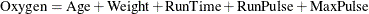The null hypothesis is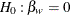, where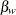is the regression parameter for the variable Weight. Suppose that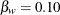is the reference improvement that should be detected at a 0.90 level. Then the maximum information
can be derived in the SEQDESIGN procedure.

Following the derivations in the section "Test for a Parameter in the Regression Model" in the chapter "The SEQDESIGN Procedure," the required sample size can be derived from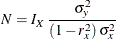where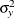is the variance of the response variable in the regression model,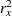is the proportion of variance of Weight explained by other covariates, and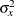is the variance of Weight.

Further suppose that from past experience,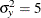,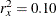, and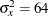. Then the required sample size can be derived using the SAMPLESIZE statement in the SEQDESIGN procedure.

The following statements invoke the SEQDESIGN procedure and request a three-stage group sequential design for normally distributed data to test the null hypothesis of a regression parameteragainst the alternative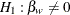:

ods graphics on;
proc seqdesign altref=0.10;
OBFErrorFunction: design method=errfuncobf
nstages=3
info=cum(2 3 4)
;
samplesize model=reg(variance=5 xvariance=64 xrsquare=0.10);
ods output Boundary=Bnd_Fit;
run;
ods graphics off;


By default (or equivalently if you specify ALPHA=0.05 and BETA=0.10), the procedure uses a Type I error probability 0.05 and a Type II error probability 0.10. The ALTREF=0.10 option specifies a power of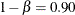at the alternative hypothesis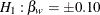. The INFO=CUM(2 3 4) option specifies that the study perform the first interim analysis with information proportion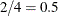—that is, after half of the total observations are collected.

The ODS OUTPUT statement with the BOUNDARY=BND_FIT option creates an output data set named BND_FIT which contains the resulting boundary information for the subsequent sequential tests.

The "Design Information" table in Output 90.2.1 displays design specifications and derived statistics. Since the alternative reference is specified, the maximum information is derived.

The SEQDESIGN Procedure
Design: OBFErrorFunction

Statistic Distribution Normal
Boundary Scale Standardized Z
Alternative Hypothesis Two-Sided
Early Stop Reject Null
Method Error Spending
Boundary Key Both
Alternative Reference 0.1
Number of Stages 3
Alpha 0.05
Beta 0.1
Power 0.9
Max Information (Percent of Fixed Sample) 101.8276
Max Information 1069.948
Null Ref ASN (Percent of Fixed Sample) 101.2587
Alt Ref ASN (Percent of Fixed Sample) 77.81586

The "Boundary Information" table in Output 90.2.2 displays information level, alternative reference, and boundary values at each stage.

Output 90.2.2: Boundary Information

Boundary Information (Standardized Z Scale)
Null Reference = 0
_Stage_   Alternative Boundary Values
Information Level Reference Lower Upper
Proportion Actual N Lower Upper Alpha Alpha
1 0.5000 534.9738 46.43869 -2.31295 2.31295 -2.96259 2.96259
2 0.7500 802.4606 69.65804 -2.83277 2.83277 -2.35902 2.35902
3 1.0000 1069.948 92.87739 -3.27101 3.27101 -2.01409 2.01409

With ODS Graphics enabled, a detailed boundary plot with the rejection and acceptance regions is displayed, as shown in Output 90.2.3. The boundary plot also displays the information level and critical value for the corresponding fixed-sample design. This design has characteristics of an O’Brien-Fleming design; the probability for early stopping is low, and the maximum information and critical values at the final stage are similar to those of the corresponding fixed-sample design.

Output 90.2.3: Boundary Plot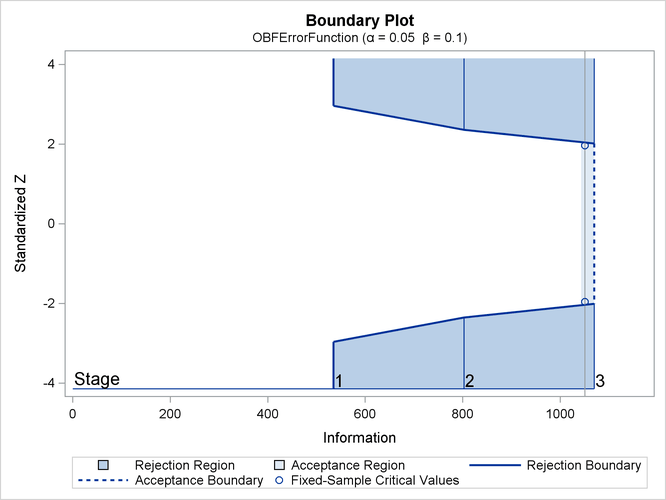With the MODEL=REG option in the SAMPLESIZE statement, the "Sample Size Summary" table in Output 90.2.4 displays the parameters for the sample size computation.

Output 90.2.4: Required Sample Size Summary

Sample Size Summary
Test Reg Parameter
Parameter 0.1
Variance 5
X Variance 64
R Square (X) 0.1
Max Sample Size 92.87739
Expected Sample Size (Null Ref) 92.35845
Expected Sample Size (Alt Ref) 70.97617

The "Sample Sizes" table in Output 90.2.5 displays the required sample sizes for the group sequential clinical trial.

Output 90.2.5: Required Sample Sizes

Sample Sizes (N)
Z Test for Regression Parameter
_Stage_ Fractional N Ceiling N
N Information N Information
1 46.44 535.0 47 541.4
2 69.66 802.5 70 806.4
3 92.88 1069.9 93 1071.4

Thus, 47, 70, and 93 individuals are needed in stages 1, 2, and 3, respectively. Since the sample sizes are derived from estimated values of,, and, the actual information levels might not achieve the target information levels. Thus, instead of specifying sample sizes in the protocol, you can specify the maximum information levels. Then if an actual information level is much less than the target level, you can increase the sample sizes for the remaining stages to achieve the desired information levels and power.

Suppose that 47 individuals are available at stage 1. Output 90.2.6 lists the first 10 observations of the trial data.

Output 90.2.6: Clinical Trial Data

 First 10 Obs in the Trial Data

Obs Oxygen Age Weight RunTime RunPulse MaxPulse
1 54.5521 44 87.7676 11.6949 178.435 181.607
2 52.2821 40 75.4853 9.8872 184.433 183.667
3 62.1871 44 89.0638 8.7950 155.540 167.108
4 65.3269 42 67.7310 8.4577 162.926 173.877
5 59.9809 37 93.1902 9.3228 179.033 180.144
6 52.5588 47 75.9044 12.0385 177.753 175.033
7 51.7838 40 73.5422 11.6607 175.838 178.140
8 57.0024 43 81.2861 11.2219 160.963 171.770
9 48.0775 44 85.2290 13.1789 173.722 176.548
10 68.3357 38 80.2490 8.5066 171.824 184.011

The following statements use the REG procedure to estimate the slopeand its associated standard error at stage 1:

proc reg data=Fit_1;
model Oxygen=Age Weight RunTime RunPulse MaxPulse;
ods output ParameterEstimates=Parms_Fit1;
run;


The following statements create and display (in Output 90.2.7) the input data set that contains slopeand its associated standard error for the SEQTEST procedure:

data Parms_Fit1;
set Parms_Fit1;
if Variable='Weight';
_Scale_='MLE';
_Stage_= 1;
keep _Scale_ _Stage_ Variable Estimate StdErr;
run;

proc print data=Parms_Fit1;
title 'Statistics Computed at Stage 1';
run;


Output 90.2.7: Statistics Computed at Stage 1

 Statistics Computed at Stage 1

Obs Variable Estimate StdErr _Scale_ _Stage_
1 Weight 0.03772 0.04345 MLE 1

The following statements invoke the SEQTEST procedure to test for early stopping at stage 1:

ods graphics on;
proc seqtest Boundary=Bnd_Fit
Parms(Testvar=Weight)=Parms_Fit1
order=lr
stopprob
;
ods output Test=Test_Fit1;
run;
ods graphics off;


The BOUNDARY= option specifies the input data set that provides the boundary information for the trial at stage 1 (this data set was generated in the SEQDESIGN procedure). Recall that these boundary values were derived for the information levels specified with the INFO=CUM(2 3 4) option in the SEQDESIGN procedure. The PARMS=PARMS_FIT1 option specifies the input data set PARMS_FIT1 that contains the test statistic and its associated standard error at stage 1, and the TESTVAR=WEIGHT option identifies the test variable WEIGHT in the data set.

If the computed information level for stage 1 is not the same as the value provided in the BOUNDARY= data set, the INFOADJ=PROP option (which is the default) proportionally adjusts the information levels at future interim stages from the levels provided in the BOUNDARY= data set. The ORDER=LR option uses the LR ordering to derive the p-value, the unbiased median estimate, and the confidence limits for the regression slope estimate. The ERRSPENDADJ=ERRFUNCOBF option adjusts the boundaries with the updated error spending values generated from an O’Brien-Fleming-type cumulative error spending function.

The ODS OUTPUT statement with the TEST=TEST_FIT1 option creates an output data set named TEST_FIT1 which contains the updated boundary information for the test at stage 1. The adjustment is needed because the observed information level is different from the information level in the BOUNDARY= data set. The data set TEST_FIT1 also provides the boundary information that is needed for the group sequential test at the next stage.

The "Design Information" table in Output 90.2.8 displays the design specifications. By default (or equivalently if you specify BOUNDARYKEY=ALPHA), the boundary values are modified for the new information levels to maintain the Type Ilevel. The maximum information remains the same as in the BOUNDARY= data set, but the derived Type II error probabilityand power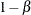are different because of the new information level.

The SEQTEST Procedure

BOUNDARY Data Set WORK.BND_FIT
Data Set WORK.PARMS_FIT1
Statistic Distribution Normal
Boundary Scale Standardized Z
Alternative Hypothesis Two-Sided
Early Stop Reject Null
Number of Stages 3
Alpha 0.05
Beta 0.09994
Power 0.90006
Max Information (Percent of Fixed Sample) 101.8057
Max Information 1069.94751
Null Ref ASN (Percent of Fixed Sample) 101.2416
Alt Ref ASN (Percent of Fixed Sample) 77.87607

With the STOPPROB option, the "Expected Cumulative Stopping Probabilities" table in Output 90.2.9 displays the expected stopping stage and cumulative stopping probability to reject the null hypothesis at each stage under various hypothetical references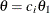, where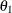is the alternative reference and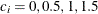by default. You can specify other values for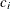with the CREF= option.

Output 90.2.9: Stopping Probabilities

Expected Cumulative Stopping Probabilities
Reference = CRef * (Alt Reference)
CRef Expected
Stopping Stage
Source Stopping Probabilities
Stage_1 Stage_2 Stage_3
0.0000 2.978 Reject Null 0.00289 0.01906 0.05000
0.5000 2.792 Reject Null 0.03373 0.17443 0.36566
1.0000 2.069 Reject Null 0.24884 0.68206 0.90006
1.5000 1.348 Reject Null 0.68172 0.97032 0.99820

The "Test Information" table in Output 90.2.10 displays the boundary values for the test statistic. By default (or equivalently if you specify BOUNDARYSCALE=STDZ), these statistics are displayed with the standardized Z scale. The information level at stage 1 is derived from the standard error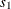in the PARMS= data set,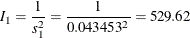Output 90.2.10: Sequential Tests

Test Information (Standardized Z Scale)
Null Reference = 0
_Stage_   Alternative Boundary Values Test
Information Level Reference Lower Upper Weight
Proportion Actual Lower Upper Alpha Alpha Estimate Action
1 0.4950 529.6232 -2.30135 2.30135 -2.97951 2.97951 0.86798 Continue
2 0.7475 799.7853 -2.82805 2.82805 -2.36291 2.36291 .
3 1.0000 1069.948 -3.27101 3.27101 -2.01336 2.01336 .

With the INFOADJ=PROP option (which is the default), the information level at stage 2 is derived proportionally from the observed information at stage 1 and the information levels in the BOUNDARY= data set. See the section Boundary Adjustments for Information Levels for details about how the adjusted information levels are computed.

At stage 1, the standardized Z statistic 0.86798 is between the lowerboundary –2.97951 and the upperboundary 2.97951, so the trial continues to the next stage.

With ODS Graphics enabled, a boundary plot with test statistics is displayed, as shown in Output 90.2.11. As expected, the test statistic is in the continuation region between the lower and upperboundaries.

Output 90.2.11: Sequential Test Plot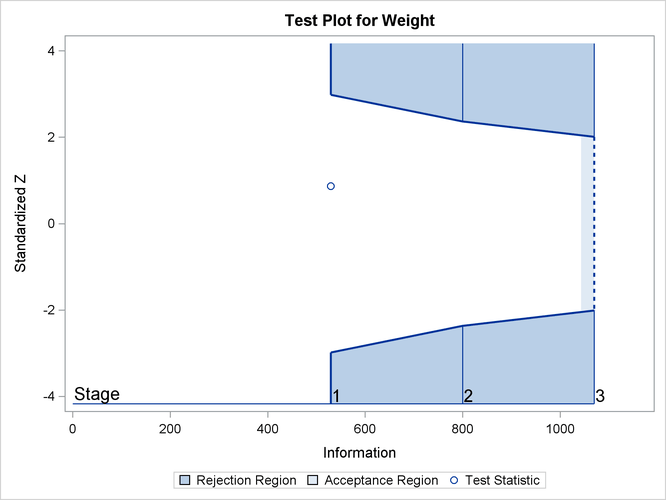The following statements use the REG procedure to estimate the slopeand its associated standard error at stage 2:

proc reg data=Fit_2;
model Oxygen=Age Weight RunTime RunPulse MaxPulse;
ods output ParameterEstimates=Parms_Fit2;
run;


Note that the data set Fit_2 contains both the data from stage 1 and the data from stage 2.

The following statements create and display (in Output 90.2.12) the input data set that contains slopeand its associated standard error at stage 2 for the SEQTEST procedure:

data Parms_Fit2;
set Parms_Fit2;
if Variable='Weight';
_Scale_='MLE';
_Stage_= 2;
keep _Scale_ _Stage_ Variable Estimate StdErr;
run;

proc print data=Parms_Fit2;
title 'Statistics Computed at Stage 2';
run;


Output 90.2.12: Statistics Computed at Stage 2

 Statistics Computed at Stage 2

Obs Variable Estimate StdErr _Scale_ _Stage_
1 Weight 0.02932 0.03520 MLE 2

The following statements invoke the SEQTEST procedure to test for early stopping at stage 2:

proc seqtest Boundary=Test_Fit1
Parms(Testvar=Weight)=Parms_Fit2
order=lr
;
ods output Test=Test_Fit2;
run;


The BOUNDARY= option specifies the input data set that provides the boundary information for the trial at stage 2, which was generated by the SEQTEST procedure at the previous stage. The PARMS= option specifies the input data set that contains the test statistic and its associated standard error at stage 2, and the TESTVAR= option identifies the test variable in the data set.

The ODS OUTPUT statement with the TEST=TEST_FIT2 option creates an output data set named TEST_FIT2 which contains the updated boundary information for the test at stage 2. The data set also provides the boundary information that is needed for the group sequential test at the next stage.

Since the data set PARMS_FIT2 does not contain the test information at stage 1, the information level at stage 1 in the TEST_FIT1 data set is used to generate boundary values for the test at stage 2.

Following the process at stage 1, the slope estimate is also between its corresponding lower and upperboundary values, so the trial continues to the next stage.

The following statements use the REG procedure to estimate the slopeand its associated standard error at the final stage:

proc reg data=Fit_3;
model Oxygen=Age Weight RunTime RunPulse MaxPulse;
ods output ParameterEstimates=Parms_Fit3;
run;


The following statements create the input data set that contains slopeand its associated standard error at stage 3 for the SEQTEST procedure:

data Parms_Fit3;
set Parms_Fit3;
if Variable='Weight';
_Scale_='MLE';
_Stage_= 3;
keep _Scale_ _Stage_ Variable Estimate StdErr;
run;


The following statements print (in Output 90.2.13) the test statistics at stage 3:

proc print data=Parms_Fit3;
title 'Statistics Computed at Stage 3';
run;


Output 90.2.13: Statistics Computed at Stage 3

 Statistics Computed at Stage 3

Obs Variable Estimate StdErr _Scale_ _Stage_
1 Weight 0.02189 0.03028 MLE 3

The following statements invoke the SEQTEST procedure to test the hypothesis:

ods graphics on;
proc seqtest Boundary=Test_Fit2
Parms(testvar=Weight)=Parms_Fit3
order=lr
pss
plots=(asn power)
;
ods output Test=Test_Fit3;
run;
ods graphics off;


The BOUNDARY= option specifies the input data set that provides the boundary information for the trial at stage 3, which was generated by the SEQTEST procedure at the previous stage. The PARMS= option specifies the input data set that contains the test statistic and its associated standard error at stage 3, and the TESTVAR= option identifies the test variable in the data set.

The ODS OUTPUT statement with the TEST=TEST_FIT3 option creates an output data set named TEST_FIT3 which contains the updated boundary information for the test at stage 3.

The "Design Information" table in Output 90.2.14 displays design specifications. By default (or equivalently if you specify BOUNDARYKEY=ALPHA), the boundary values are modified for the new information levels to maintain the Type Ilevel.

The SEQTEST Procedure

BOUNDARY Data Set WORK.TEST_FIT2
Data Set WORK.PARMS_FIT3
Statistic Distribution Normal
Boundary Scale Standardized Z
Alternative Hypothesis Two-Sided
Early Stop Reject Null
Number of Stages 3
Alpha 0.05
Beta 0.09514
Power 0.90486
Max Information (Percent of Fixed Sample) 102.0102
Max Information 1090.63724
Null Ref ASN (Percent of Fixed Sample) 101.4122
Alt Ref ASN (Percent of Fixed Sample) 77.22139

The maximum information is derived from the standard error associated with the slope estimate at the final stage and is larger than the target level. The derived Type II error probabilityand powerare different because of the new information levels.

With the PSS option, the "Power and Expected Sample Sizes" table in Output 90.2.15 displays powers and expected mean sample sizes under various hypothetical references, whereis the alternative reference andby default. You can specify thevalues with the CREF= option.

Output 90.2.15: Power and Expected Sample Size Information

Powers and Expected Sample Sizes
Reference = CRef * (Alt Reference)
CRef Power Sample Size
Percent
Fixed-Sample
0.0000 0.02500 101.4122
0.5000 0.37046 96.3754
1.0000 0.90486 77.2214
1.5000 0.99844 58.5301

With the PLOTS=ASN option, the procedure displays a plot of expected sample sizes under various hypothetical references, as shown in Output 90.2.16. By default, expected sample sizes under the hypotheses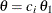,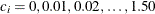, are displayed, whereis the alternative reference.

Output 90.2.16: ASN Plot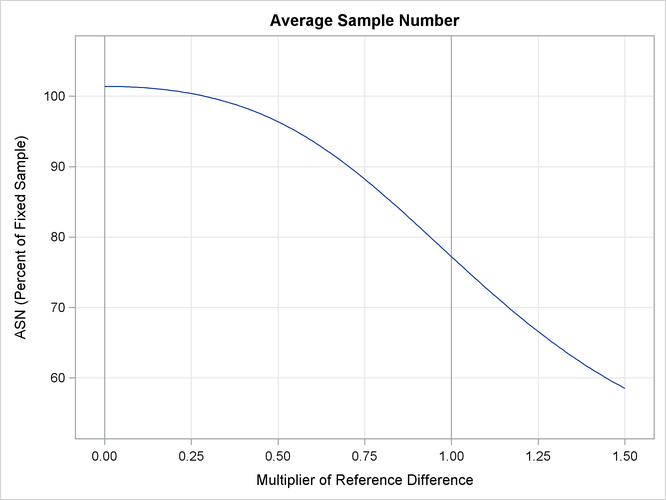With the PLOTS=POWER option, the procedure displays a plot of powers under various hypothetical references, as shown in Output 90.2.17. By default, powers under hypothetical referencesare displayed, whereby default. You can specifyvalues with the CREF= option. Thevalues are displayed on the horizontal axis.

Output 90.2.17: Power Plot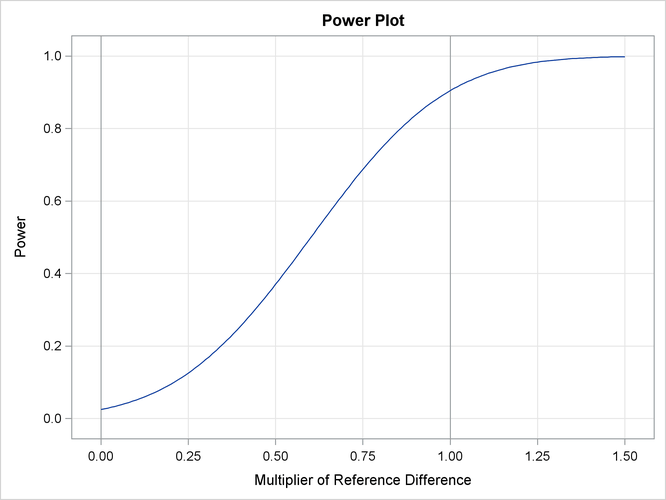Under the null hypothesis,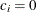, the power is 0.025, which is the upper Type I error probability. Under the alternative hypothesis,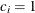, the power is 0.90486, which is one minus the Type II error probability.

The "Test Information" table in Output 90.2.18 displays the boundary values for the test statistic with the default standardized Z scale. The information level at the current stage is derived from the standard error for the current stage in the PARMS= data set. At stage 3, the standardized slope estimate 0.72284 is still between the lower and upperboundary values. Since it is the final stage, the trial stops to accept the null hypothesis that the variable Weight has no effect on the oxygen intake rate after adjusting for other covariates.

Output 90.2.18: Sequential Tests

Test Information (Standardized Z Scale)
Null Reference = 0
_Stage_   Alternative Boundary Values Test
Information Level Reference Lower Upper Weight
Proportion Actual Lower Upper Alpha Alpha Estimate Action
1 0.4856 529.6232 -2.30135 2.30135 -2.97951 2.97951 0.86798 Continue
2 0.7401 807.1954 -2.84112 2.84112 -2.34945 2.34945 0.83305 Continue
3 1.0000 1090.637 -3.30248 3.30248 -2.01885 2.01885 0.72284 Accept Null

Since the data set FIT_3 contains the test information only at stage 3, the information levels at previous stages in the TEST_FIT2 data set are used to generate boundary values for the test.

With ODS Graphics enabled, a boundary plot with test statistics is displayed, as shown in Output 90.2.19. As expected, the test statistic is in the acceptance region between the lower and upperboundaries at the final stage.

Output 90.2.19: Sequential Test Plot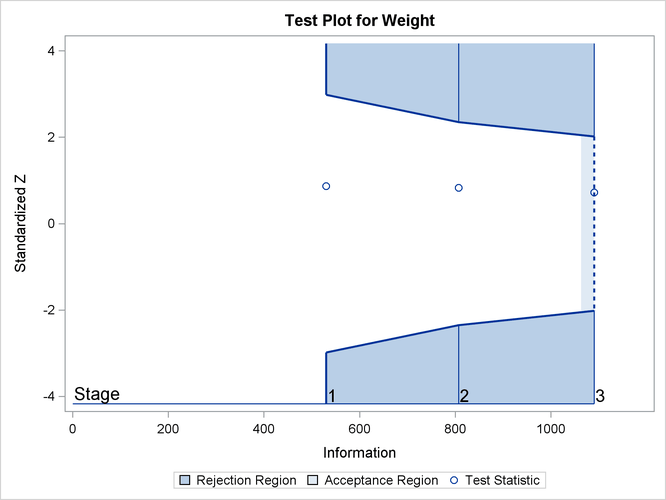After a trial is stopped, the "Parameter Estimates" table in Output 90.2.20 displays the stopping stage, parameter estimate, unbiased median estimate, confidence limits, and the p-value under the null hypothesis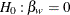.

Output 90.2.20: Parameter Estimates

Parameter Estimates
LR Ordering
Parameter Stopping
Stage
MLE p-Value for
H0:Parm=0
Median
Estimate
95% Confidence Limits
Weight 3 0.021888 0.4699 0.021884 -0.03747 0.08123

As expected, the p-value 0.4699 is not significant at the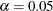level, and the confidence interval does contain the value zero. The p-value, unbiased median estimate, and confidence limits depend on the ordering of the sample space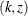, where k is the stage number and z is the standardized Z statistic. With the specified LR ordering, the p-values are computed with the orderingif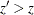. See the section Available Sample Space Orderings in a Sequential Test for a detailed description of the LR ordering.